Next: Range Up: Azimuth Previous: line number   Contents

### Doppler centroid

The Doppler centroid frequency (azimuth) is computed as a second degree polynomial: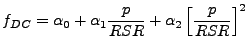(D34)

Where:
p is the pixel number starting at 0.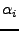is read from the leader file.
In the master result file it are the variables (e.g.):
 Xtrack_f_DC_constant (Hz, early edge):              117.3210000
Xtrack_f_DC_linear (Hz/s, early edge):            72338.0000000


This frequency is used in the azimuth filtering, and in the resampling. It should also be used if the complex SLC data is harmonically oversampled (as is done in the range filtering routine), but we did not implement this yet. But, for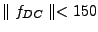Hz this should not have any effect (assuming PRF-ABW=300Hz).

Since for a signal f(t) with Fourier transform F(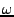)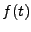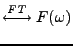(D35)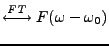(D36)

The azimuth spectrum of a SLC image processed on a certain Doppler frequency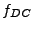(spectrum shifted to this frequency) can be shifted back to zero by multiplication in the space domain by the term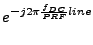(D37)

(See also any signals and systems book, or e.g., .) The spectrum can be shifted back to the original doppler centroid frequency by multiplication by (after e.g., interpolation):(D38)

Proper care should be taken to get the correct line number in both situations.Next: Range Up: Azimuth Previous: line number   Contents
Leijen 2009-04-14